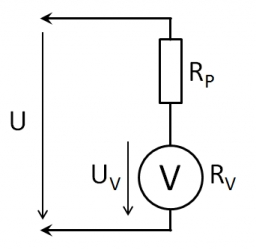# Voltmeter range

We have a voltmeter which in the original set measures voltage to 10V. Calculate the size of the ballast resistor for this voltmeter, if we want to measure the voltage up to 50V. Voltmeter's internal resistance is 2 kiloohm/Volt.

Result

R2 =  80000 Ω

#### Solution:

$U_{ 1 } = 10 \ V \ \\ U_{ 2 } = 50 \ V \ \\ \ \\ R_{ 1 } = 2 \cdot \ 1000 \cdot \ U_{ 1 } = 2 \cdot \ 1000 \cdot \ 10 = 20000 \ Ω \ \\ U_{ 3 } = U_{ 2 }-U_{ 1 } = 50-10 = 40 \ V \ \\ \ \\ I = \dfrac{ U_{ 1 } }{ R_{ 1 } } = \dfrac{ 10 }{ 20000 } = \dfrac{ 1 }{ 2000 } = 0.0005 \ A \ \\ U_{ 3 } = I \cdot \ R_{ 2 } \ \\ \ \\ R_{ 2 } = U_{ 3 }/I = 40/0.0005 = 80000 = 80000 \ \text { Ω }$Our examples were largely sent or created by pupils and students themselves. Therefore, we would be pleased if you could send us any errors you found, spelling mistakes, or rephasing the example. Thank you!

Leave us a comment of this math problem and its solution (i.e. if it is still somewhat unclear...):Be the first to comment!## Next similar math problems:

1. TV transmitterThe volume of water in the rectangular swimming pool is 6998.4 hectoliters. The promotional leaflet states that if we wanted all the pool water to flow into a regular quadrangle with a base edge equal to the average depth of the pool, the prism would have.
2. Trapezoid MOThe rectangular trapezoid ABCD with right angle at point B, |AC| = 12, |CD| = 8, diagonals are perpendicular to each other. Calculate the perimeter and area of ​​the trapezoid.
3. Axial sectionAxial section of the cone is an equilateral triangle with area 168 cm2. Calculate the volume of the cone.
4. RiverCalculate how many promiles river Dunaj average falls, if on section long 957 km flowing water from 1454 m AMSL to 101 m AMSL.
5. CuboidCuboid with edge a=6 cm and body diagonal u=31 cm has volume V=900 cm3. Calculate the length of the other edges.
6. ServerCalculate how many average minutes a year is a webserver is unavailable, the availability is 99.99%.
7. Triangle SASCalculate the area and perimeter of the triangle, if the two sides are 51 cm and 110 cm long and angle them clamped is 130 °.
8. PoolIf water flows into the pool by two inlets, fill the whole for 8 hours. The first inlet filled pool 6 hour longer than second. How long pool take to fill with two inlets separately?
9. BonusGross wage was 527 EUR including 16% bonus. How many EUR were bonuses?
10. LogicA man can drink a barrel of water for 26 days, woman for 48 days. How many days will a barrel last between them?
11. Cube in a sphereThe cube is inscribed in a sphere with volume 7253 cm3. Determine the length of the edges of a cube.
12. BeerAfter three 10° beers consumed in a short time, there is 5.6 g of alcohol in 6 kg adult human blood. How much is it per mille?
13. Circle chordWhat is the length d of the chord circle of diameter 51 mm, if the distance from the center circle is 19 mm?
14. RectangleThe rectangle is 21 cm long and 38 cm wide. Determine the radius of the circle circumscribing rectangle.
15. Trigonometric functionsIn right triangle is: ? Determine the value of s and c: ? ?
16. ClockHow many times a day hands on a clock overlap?
17. Tetrahedral pyramidWhat is the surface of a regular tetrahedral (four-sided) pyramid if the base edge a=16 and height v=16?